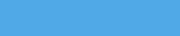# Bluish Coder

Programming Languages, Martials Arts and Computers. The Weblog of Chris Double.

2007-11-28

## Embedded Grammars in Factor

I'm working on a new parser combinator library for Factor based on Parsing Expression Grammars and Packrat parsers. This is based on what I learnt from writing a packrat parser in Javascript.

It's progressing quite well and already fixes some problems in the existing parser combinator library I wrote. The main issue with that one is it's not tail recursive and some combinations of parsers can run out of call stack.

The new library is in the 'peg' vocabulary. I haven't yet implemented the packrat side of things though so it is slow on large grammars and inputs.

I've also done a proof of concept of something I've been meaning to do for awhile. That is writing a parser for EBNF (or similar) that produces Factor code in the form of parser combinators to implement the described grammar. The code for this is in the 'peg.ebnf' vocabulary. It allows you to embed an EBNF-like language and have Factor words generated for each rule:

``````<EBNF
digit  = '1' | '2' | '3' | '4' .
number = digit { digit } .
expr   = number {('+' | '-' ) number } .
EBNF>
``````

This example would create three Factor words. 'digit', 'number' and 'expr'. These words return parsers that can be used as normal:

``````"123" number parse
"1" digit parse
"1+243+342" expr parse
``````

The EBNF accepted allows for choice, zero or more repetition, optional (exactly 0 or 1), and grouping. The generated AST is pretty ugly so by default it works best as a syntax checker. You can modify the generated AST with action productions:

``````<EBNF
digit  = '1' | '2' | '3' | '4' => convert-to-digit .
number = digit { digit }       => convert-to-number .
expr   = number '+' number     => convert-to-expr .
EBNF>
``````

An action is a factor word after the '=>'. The word receives the AST produced from the rule on the stack and it can replace that with a new value that will be used in the AST. So 'convert-to-expr' above might produce a tuple holding the expression values (by default, a sequence of terms in the rule are stored in a vector):

``````TUPLE: ast-expr lhs operator rhs ;
C: <ast-expr> ast-expr
: convert-to-expr ( old -- new )
first3 <ast-expr> ;
``````

The generated code is currently pretty ugly, mainly due to it being a quick proof of concept. I'll try doing a few grammars and tidy it up, changing the interface if needed, as I go along.

As an experiment I did a grammar for the PL/0 programming language. It's in 'peg.pl0'. The grammar from the wikipedia article is:

``````program = block "." .

block = [ "const" ident "=" number {"," ident "=" number} ";"]
[ "var" ident {"," ident} ";"]
{ "procedure" ident ";" block ";" } statement .

statement = [ ident ":=" expression | "call" ident |
"begin" statement {";" statement } "end" |
"if" condition "then" statement |
"while" condition "do" statement ].

condition = "odd" expression |
expression ("="|"#"|"<"|"<="|">"|">=") expression .

expression = [ "+"|"-"] term { ("+"|"-") term}.

term = factor {("*"|"/") factor}.

factor = ident | number | "(" expression ")".</pre>The Factor grammar is very similar:<pre>: ident ( -- parser )
CHAR: a CHAR: z range
CHAR: A CHAR: Z range 2array choice repeat1
[ >string ] action ;

: number ( -- parser )
CHAR: 0 CHAR: 9 range repeat1 [ string>number ] action ;

<EBNF
program = block '.' .
block = [ 'const' ident '=' number { ',' ident '=' number } ';' ]
[ 'var' ident { ',' ident } ';' ]
{ 'procedure' ident ';' [ block ';' ] } statement .
statement = [ ident ':=' expression | 'call' ident |
'begin' statement {';' statement } 'end' |
'if' condition 'then' statement |
'while' condition 'do' statement ] .
condition = 'odd' expression |
expression ('=' | '#' | '<=' | '<' | '>=' | '>') expression .
expression = ['+' | '-'] term {('+' | '-') term } .
term = factor {('*' | '/') factor } .
factor = ident | number | '(' expression ')'
EBNF>
``````

This grammar as defined works and can parse PL/0 programs. I'll extend this as I improve the EBNF routines, adding actions, etc to generated a decent AST.

### Tags

This site is accessable over tor as hidden service mh7mkfvezts5j6yu.onion, or Freenet using key:
USK@1ORdIvjL2H1bZblJcP8hu2LjjKtVB-rVzp8mLty~5N4,8hL85otZBbq0geDsSKkBK4sKESL2SrNVecFZz9NxGVQ,AQACAAE/bluishcoder/-61/

Tags

Archives